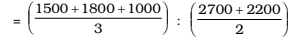## Introduction to Data Interpretation

#### Data Interpretation

Direction: Study the graph and answer the following questions.1. The average production of the companies A, B, C and that of the companies D, E are in the ratio :

1. From the given bar diagram , we see
Total production of the companies A, B and C = 1500 + 1800 + 1000
Number of companies A, B and C = 3
Total production of the companies D and E = 2700 + 2200
Number of companies D and E = 2
Required ratio = Average production of the companies A, B and C : Average production of the companies D and E

##### Correct Option: B

From the given bar diagram , we see
Total production of the companies A, B and C = 1500 + 1800 + 1000
Number of companies A, B and C = 3
Total production of the companies D and E = 2700 + 2200
Number of companies D and E = 2
Required ratio = Average production of the companies A, B and C : Average production of the companies D and E
Required ratioRequired ratio = 2 × 4300 : 4900 × 3 = 86 : 147

1. What is the difference between average demand and average production of the companies taken together?

1. On the basis of given graph in question ,
Total demand of the companies = 3000 + 600 + 2500 + 1200 + 3300 = 10600
Number of companies = 5

 ∴ Average demand = Total demand of the companies Number of companies

 ∴ Average demand = 10600 = 2120 5

Total production of the companies = 1500 + 1800 + 1000 + 2700 + 2200 = 9200
 ∴ Average production = Total production of the companies Number of companies

##### Correct Option: C

On the basis of given graph in question ,
Total demand of the companies = 3000 + 600 + 2500 + 1200 + 3300 = 10600
Number of companies = 5

 ∴ Average demand = Total demand of the companies Number of companies

 ∴ Average demand = 10600 = 2120 5

Total production of the companies = 1500 + 1800 + 1000 + 2700 + 2200 = 9200
 ∴ Average production = Total production of the companies Number of companies

 ∴ Average production = 10600 = 1840 5

Required difference = Average demand : Average production
Required difference = 2120 – 1840 = 280

1. What percent of the demand of company C is that of the company B?

1. According to given bar graph , we have
The demand of company C = 2500 units
The demand of company B = 600 units

 Required percentage = The demand of company B × 100 The demand of company C

##### Correct Option: C

According to given bar graph , we have
The demand of company C = 2500 units
The demand of company B = 600 units

 Required percentage = The demand of company B × 100 The demand of company C

 Required percentage = 600 × 100 = 24% 25001. Which of the above States is the largest producer of wheat?

1. As per the given bar graph , we have
Uttar Pradesh (UP) produces 16 million tonnes of wheat that is largest.

##### Correct Option: D

As per the given bar graph , we have
Uttar Pradesh (UP) produces 16 million tonnes of wheat that is largest. Hence , required answer is Uttar Pradesh (UP) .

1. In which of the above states the total production of rice and wheat is the least?

1. According to given bar graph , we have
Production of rice least in Maharashtra = 3 million tonnes
Production of wheat least in Maharashtra = 2 million tonnes

##### Correct Option: C

According to given bar graph , we have
Production of rice least in Maharashtra = 3 million tonnes
Production of wheat least in Maharashtra = 2 million tonnes
Total production of rice and wheat is least in Maharashtra 5 million tonnes .Hence , required answer is Maharashtra .